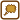## Movement of cestre of mass of a mass point system

Three mass points with masses of 5 g, 10 g and 15 g make up a mass point system. At time t0 = 0 s, all points are at rest in positions A1 = [10, 4, 6] cm, A2 = [-2, 4, -9] cm, A3 = [0, 0, 0] cm.

These mass points start to move because of external forces, whose resultant has value of 0,06 N and both direction and orientation of the x-axis. Find the location of the centre of mass of this mass point system at time t1 = 2 s.

• #### List of known information

 m1 = 5 g mass of 1st mass point m2 = 10 g mass of 2nd mass point m3 = 15 g mass of 3rd mass point A1 = [10, 4, 6] cm starting position of 1st mass point A2 = [-2, 4, -9] cm starting position of 2nd mass point A3 = [0, 0, 0] cm starting position of 3rd mass point t0 = 0 s initial time v0 = 0 m·s−1 initial velocity of mass points t1 = 2 s final time F = 0,06 N resultant of forces with both direction and orientation of the x-axis rT(t1 ) = ? position of centre of mass at time t1
• #### Analysis

During the solving of the task, we first determine the initial position of the centre of mass T0 of the system. For all other calculations, the mass is concentrated at this centre and if we want to know how the external force affects the movement of the system, we will only need to find out the movement of the centre of mass T0.

• #### Hint 1

To find the final position of the centre of mass T1 after 2 seconds of movement, we first need to know its initial position T0. Do you know the relation for the location of the centre of mass of a mass point system? Use it.

• #### Hint 2

Now we make the system move because of the acting of constant force F in the direction of the x-axis. Will all of the coordinates of the centre of mass change T0 change?

• #### Hint 3

The movement of the whole system (and thus its centre of mass as well) is realised by the acting of a constant force F. What type of movement is this (in terms of the change in velocity)? Newton’s second law will help you.

• #### Hint 4

The increase of the x coordinate of the centre of mass can be determined as displacement that the centre of mass travels during this type of motion in given time. Substitute numerical values and calculate the final position of the centre of mass.

• #### Overall Solution

First, we determine the initial coordinates of the centre of mass of the system. The relation for the centre of mass of a system of  n mass points is given as:

$\vec{r}_T\,=\,\frac{\sum_{i=1}^n{m_i\vec{r}_i}}{\sum_{i=1}^n{m_i}},$

where mi are the masses of individual mass points and ri are their position vectors. This applies for individual parts because the coordinates of the centre of mass is calculable by parts:

$x_T\,=\,\frac{\sum_{i=1}^n{m_ix_i}}{\sum_{i=1}^n{m_i}},\tag{1}$ $y_T\,=\,\frac{\sum_{i=1}^n{m_iy_i}}{\sum_{i=1}^n{m_i}},\tag{2}$ $z_T\,=\,\frac{\sum_{i=1}^n{m_iz_i}}{\sum_{i=1}^n{m_i}}.\tag{3}$

In our case, we will substitute masses and coordinates of points A1 through A3into relations (1) through (3). This way, we will calculate the initial position of the centre of mass T0:

$x_{T_0}\,=\,\frac{\sum_{i=1}^3{m_ix_i}}{\sum_{i=1}^3{m_i}}\,=\,\frac{5{\cdot}10\,+\,10{\cdot}(-2)\,+\,15{\cdot}0}{5\,+\,10\,+\,15}\,\mathrm{cm}\,=\,1\,\mathrm{cm},$ $y_{T_0}\,=\,\frac{\sum_{i=1}^3{m_iy_i}}{\sum_{i=1}^3{m_i}}=\,\frac{5{\cdot}4\,+\,10{\cdot}4\,+\,15{\cdot}0}{5\,+\,10\,+\,15}\,\mathrm{cm}\,=\,2\,\mathrm{cm},$ $z_{T_0}\,=\,\frac{\sum_{i=1}^3{m_iz_i}}{\sum_{i=1}^3{m_i}}=\,\frac{5{\cdot}6\,+\,10{\cdot}(-9)\,+\,15{\cdot}0}{5\,+\,10\,+\,15}\,\mathrm{cm}\,=\,-2\,\mathrm{cm}.$

The initial position of the centre of mass is thus T0 =  [1, 2, -2]  cm.

The movement of the system in the direction of the x-axis is changing only its x coordinates of the mass points so only the x coordinates of the centre of mass. The initial coordinates yT0 and zT0 aren’t changing during the movements, so the final coordinates of the centre of mass T1 will be:

$y_{T_1}\,=\,y_{T_0}\,=\,2\,\mathrm{cm},$ $z_{T_1}\,=\,z_{T_0}\,=\,-2\,\mathrm{cm}.$

Now we only need to determine the final xT1 coordinate of the centre of mass.

According to Newton’s 2nd law, the following applies to our system:

$F\,=\,ma\,=\,(m_1\,+\,m_2\,+\,m_3)a\Rightarrow\,a\,=\,\frac{F}{m_1\,+\,m_2\,+\,m_3},\tag{4}$

where F is the force acting on our system, m is its total mass (here, evidently m = m1 + m2 + m3) and a is the acceleration of the system’s centre of mass. The expression on the right side of relation (4) for the constant force F is also a constant; the movement is thus evenly accelerated. During this movement with acceleration a and null initial velocity, we can determine the increase of the x coordinate of the centre of mass as displacement Δx that the centre of mass travels because of the acting of the force F over time t1 − t0  like this:

${\Delta}x\,=\,\frac{1}{2}a(t_1\,-\,t_0)^2.\tag{5}$

With the help of relation (4), we will adjust relation (5):

${\Delta}x\,=\,\frac{1}{2}\frac{F}{m_1\,+\,m_2\,+\,m_3}(t_1\,-\,t_0)^2.\tag{6}$

We can then get the final x coordinate of the centre of mass xT1 as a sum of the initial coordinate xT0 and the change Δx:

$x_{T_1}\,=\,x_{T_0}\,+\,{\Delta}x.\tag{7}$

Substituting into the relation (6) gives us the final equation:

$x_{T_1}\,=\,x_{T_0}\,+\,\frac{1}{2}\frac{F}{m_1\,+\,m_2\,+\,m_3}(t_1\,-\,t_0)^2.\tag{8}$

Numerically:

$x_{T_1}\,=(\,0{,}01\,+\,\frac{1}{2}\frac{0{,}06}{0{,}005\,+\,0{,}010\,+\,0{,}015}(2\,-\,0)^2)\,\mathrm{m}\,=\,401\,\mathrm{cm}.$

The final position of the centre of mass of the system is T1 =  [401, 2, -2]  cm.